Ex 9.2

Chapter 9 Class 11 Sequences and Series
Serial order wise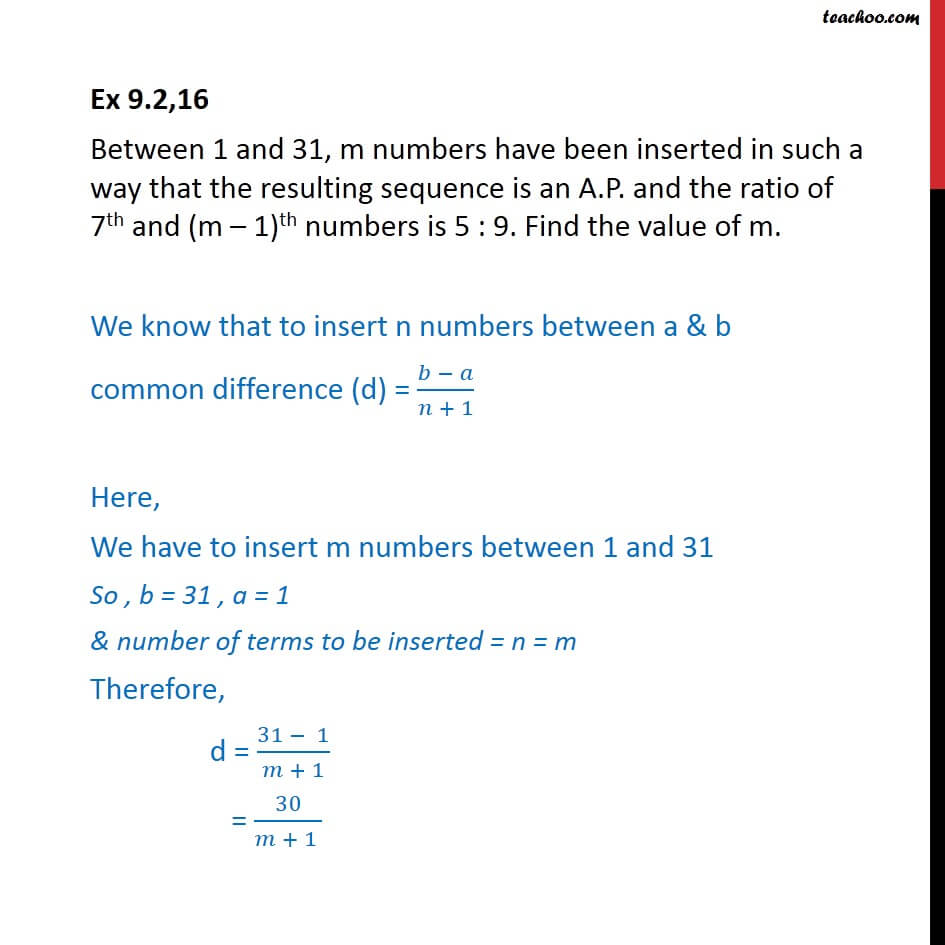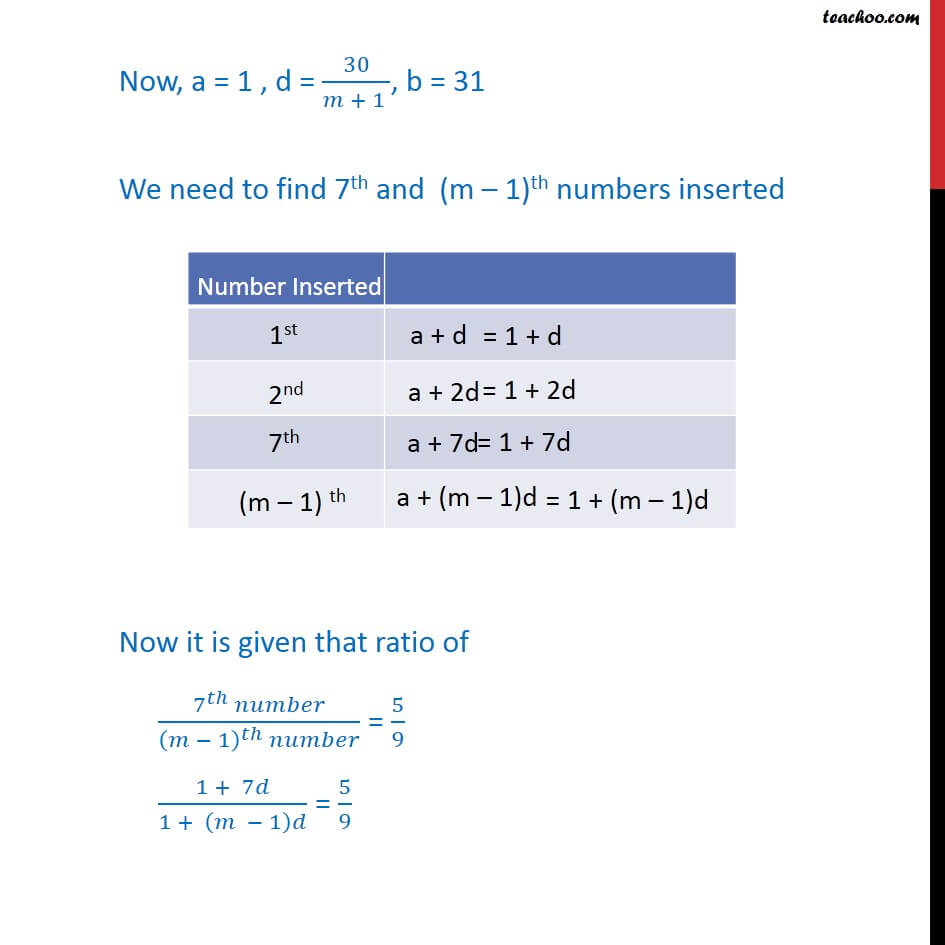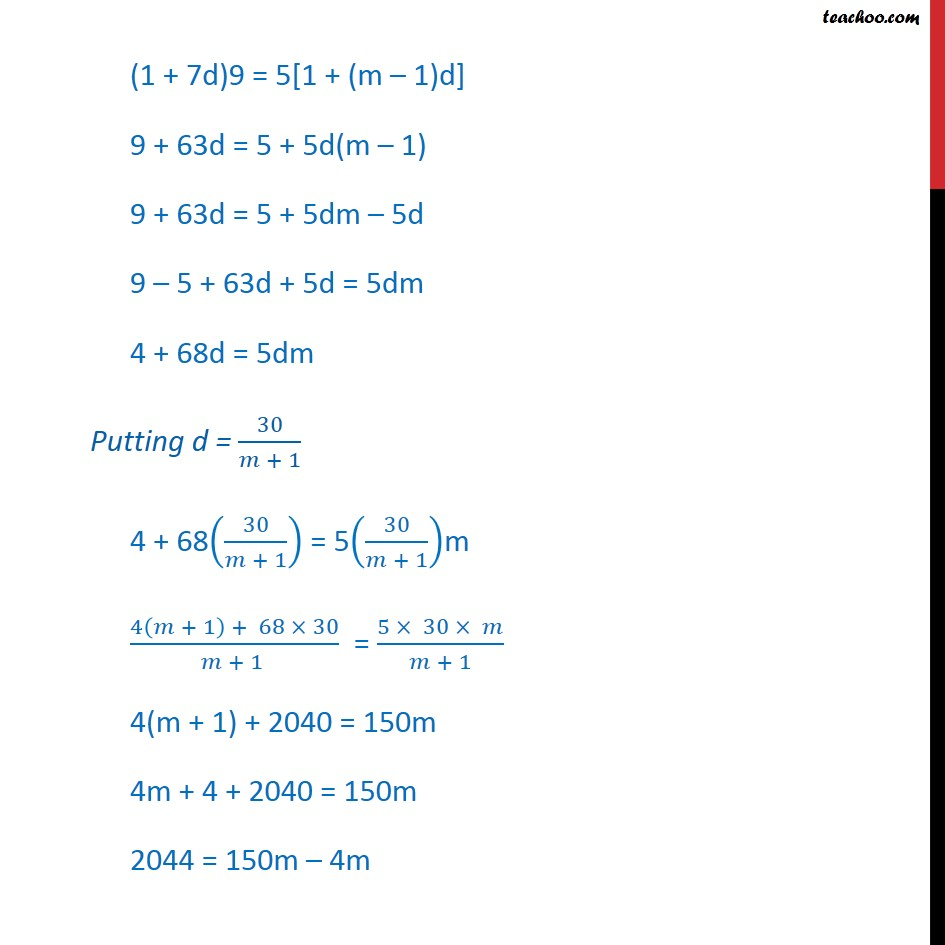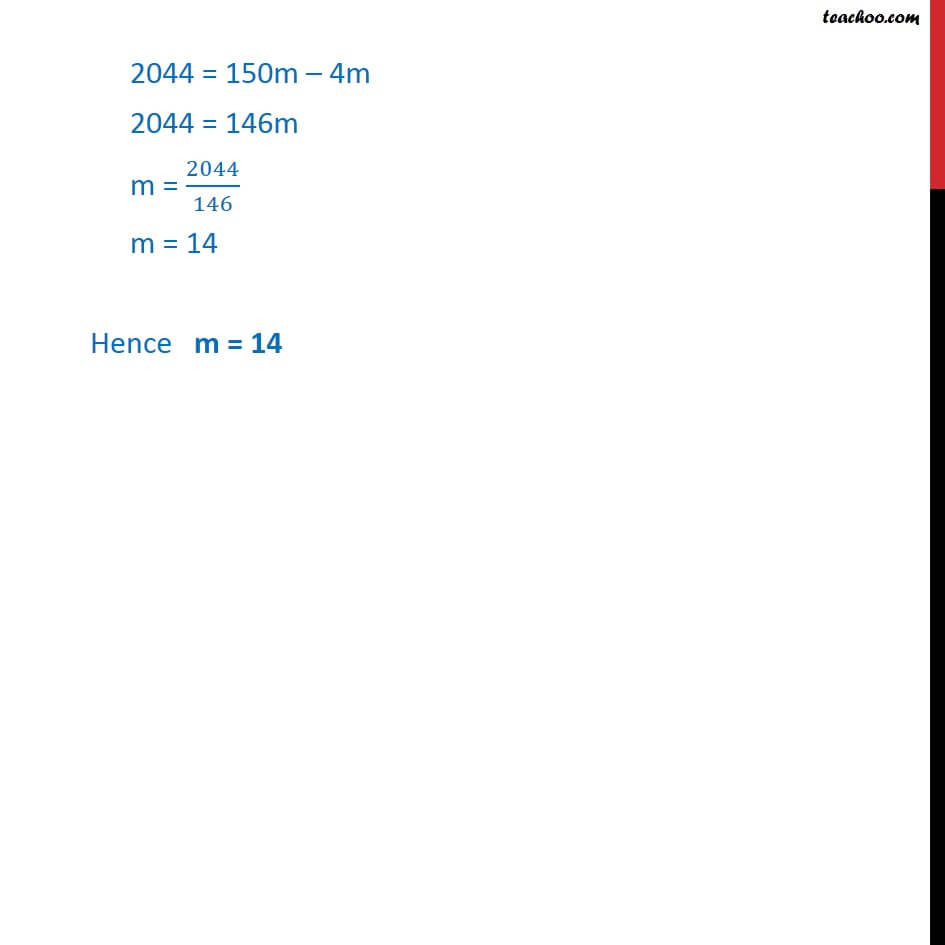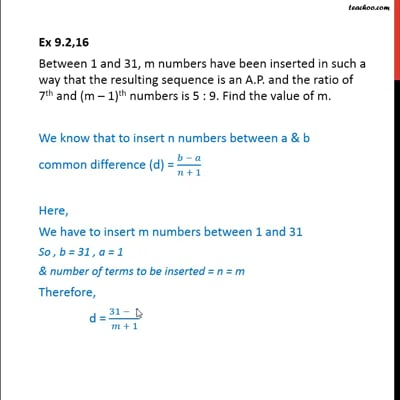This video is only available for Teachoo black users

Solve all your doubts with Teachoo Black (new monthly pack available now!)

### Transcript

Ex 9.2,16 Between 1 and 31, m numbers have been inserted in such a way that the resulting sequence is an A.P. and the ratio of 7th and (m – 1)th numbers is 5 : 9. Find the value of m. We know that to insert n numbers between a & b common difference (d) = (𝑏 − 𝑎)/(𝑛 + 1) Here, We have to insert m numbers between 1 and 31 So , b = 31 , a = 1 & number of terms to be inserted = n = m Therefore, d = (31 − 1)/(𝑚 + 1) = 30/(𝑚 + 1 ) Now, a = 1 , d = 30/(𝑚 + 1 ), b = 31 We need to find 7th and (m – 1)th numbers inserted Now it is given that ratio of (7^𝑡ℎ 𝑛𝑢𝑚𝑏𝑒𝑟)/((𝑚 − 1)^𝑡ℎ 𝑛𝑢𝑚𝑏𝑒𝑟) = 5/9 (1 + 7𝑑)/(1 + (𝑚 − 1)𝑑) = 5/9 (1 + 7𝑑)/(1 + (𝑚 − 1)𝑑) = 5/9 (1 + 7d)9 = 5[1 + (m – 1)d] 9 + 63d = 5 + 5d(m – 1) 9 + 63d = 5 + 5dm – 5d 9 – 5 + 63d + 5d = 5dm 4 + 63d = 5dm Putting d = 30/(𝑚 + 1) 4 + 63(30/(𝑚 + 1)) = 5(30/(𝑚 + 1))m (4(𝑚 + 1) + 63 × 30)/(𝑚 + 1 ) = (5 × 30 × 𝑚)/(𝑚 + 1) 4(m + 1) + 2040 = 150m 4m + 4 + 2040 = 150m 2044 = 150m – 4m 2044 = 150m – 4m 2044 = 146m m = 2044/146 m = 14 Hence m = 14